### 2.1.3 Energy Gaps and General Band StructureElectron waves with wave vectors on or near a BZ edge are diffracted; all others aren't.This means simply that electrons with wave vectors near or at a BZ edge – let's call them kBZ electrons – feel the periodic potential of the crystal while the others do not (in a first approximation).In other words, kBZ electrons interact with the crystal, and this must express itself in their energies.

Origin of Energy GapsIntuitively, we expect that "normal" electrons, not feeling any diffraction, pretty much obey the relation for the total energy E as before:
E  =  Ekin   =  p2
2m
=  (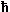k)2
2mFor kBZ electrons, however, we must expect major modifications.What we will get in the most general terms is a splitting of the energy value if a given k ends exactly at the Brillouin zone edge, i.e. for a kBZ electron. Instead of E(k) = (k)2/2me we obtain.
E(k BZ)  =  (kBZ)2
2m
±   DEIn words: Electrons at the BZ edge can have two energies for the same wave vector and thus state. One value is somewhat lower than the free electron gas value, the other one is somewhat higher.Energies between these values are unobtainable for any electron – there is now an energy gap in the E = E(k) relation for all k vectors ending on a Brillouin zone wall.The time-honored way to visualize this energy gap is to look at a one-dimensional crystal – i.e. a chain of atoms , periodically spaced with the distance a.Since in this case, we have for the electron wave meeting the Bragg condition ...
k'  =  k  =  kBZ... the electron wave will be reflected back on itself. Therefore, the solutions of the Schrödinger equation will be described by the possible superpositions of the two waves, and there are two possibilities to do that:
y+  =  1 1/2 2L æ ç è ö ÷ ø æ è ö ø

  =  1 2L 1/2 y – æ ç è ö ÷ ø æ è ö øFor the first Brillouin zone, we have g1 = 2 p/a = kk' = 2kBZ, and so k BZ = p/a. Since this can be easily generalized for higher Brillouin zones, the same consequences will occur also there. To understand the basics, however, it is sufficient to consider just the edge of the first Brillouin zone.Both solutions are no longer propagating plane waves with y · y* = const. throughout the crystal but standing waves ...
|y +|2  = æ ç è ö ÷ ø

|y  = –|2 æ ç è ö ÷ ø... with the maxima being at the coordinates of the atoms for the y+ solution and between the atoms for the y solution.In the first case the potential energy of the electrons is lowered , in the second case it is raised – there is an energy gap!Note that now a potential energy is involved, but only because we now implicitly assumed that the potential is no longer constant.While this is a relatively painless way to envision the occurrence of an energy gap, the three-dimensional case needs a few more considerations.Waves with k » kBZ, while diffracted, do not have to directly run back in themselves – after some more reflections, however, they will.This leads to a splitting of the energy for all positions on the Brillouin zone edges; the amount of splitting, however, may differ .A general relation yields for the energies of the kBZ electron waves
E(k BZ)  =  (kBZ)2
2m
±   | U(g)|With U(g) = Fourier component of the periodic potential for the reciprocal lattice vector g relevant for the kBZ vector considered.

Representation of Energy Gaps and Band StructuresBearing this in mind, we now can construct the E(k) diagram in a principal way: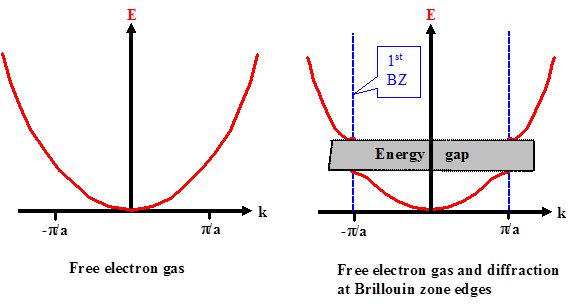In different directions we still would have an energy gap, but at different positions on the energy axis and with a different width. Nevertheless, this is already the first step to understand the electronic band structure of crystalline solids.That is about as far as the free electron gas model with diffraction added (and therefore by necessity some unspecified periodic potential) will get us.For more insights we will actually have to solve the Schrödinger equation for some kind of periodic potential. This is difficult, even for very simple (unrealistic) periodic potentials; cf. the link.For this we first need a halfway realistic potential – e.g. for a Si or a GaAs crystal – which we then use in the Schrödinger equation. The solutions will depend on the precise kind of potential and, as we must expect, they will not be easy to find (or even to express in closed form).However, since the potential is periodic, which means it doesn't matter if we look at it at r or at r + R with R = any translation vector of the lattice – it always looks the same – we may confidently expect that the solutions mirror somehow this property. After all, it should not matter much either, at which crystallographically equivalent crystal positions we look at the electrons.This is exactly what the celebrated Bloch theorem asserts: No matter what kind of periodic potential is plucked into the Schrödinger equation, the solutions must have certain properties which can be specified in a very general way.We will deal with this in the next subchapter.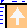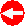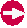© H. Föll (Semiconductors - Script)